Courses

# Test: Trusses -1

## 10 Questions MCQ Test Structural Analysis | Test: Trusses -1

Description
This mock test of Test: Trusses -1 for Civil Engineering (CE) helps you for every Civil Engineering (CE) entrance exam. This contains 10 Multiple Choice Questions for Civil Engineering (CE) Test: Trusses -1 (mcq) to study with solutions a complete question bank. The solved questions answers in this Test: Trusses -1 quiz give you a good mix of easy questions and tough questions. Civil Engineering (CE) students definitely take this Test: Trusses -1 exercise for a better result in the exam. You can find other Test: Trusses -1 extra questions, long questions & short questions for Civil Engineering (CE) on EduRev as well by searching above.
QUESTION: 1

### A simply supported truss shown in the given figure carries load as shown. The force in member BE is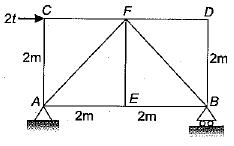Solution:

At joint D, there is no external force so force in FD and BD is zero.
Taking moment about A, the reaction at B,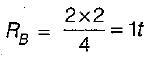Considering joint B, force in member BF,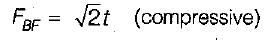force in member BE,
FBE=1t (tensile)

QUESTION: 2

Solution:
QUESTION: 3

### The force in member FD in the given figure is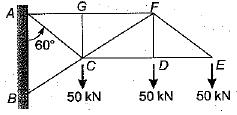Solution:

Considering joint equilibrium at D. The force equilibrium equation in vertical direction gives FD = 50 kN tensile

QUESTION: 4

In the cantilever truss shown in the given figure. the reaction at A is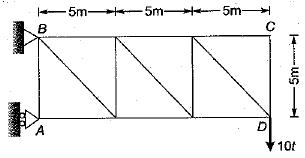Solution:

Only a horizontal reaction can exist at A.

HA x 5 = 10 x 15
HA = 30t

QUESTION: 5

Force in the member BC of the truss shown in the given figure is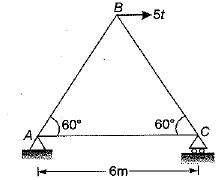Solution:

By considering joint equilibrium at B,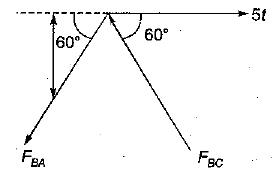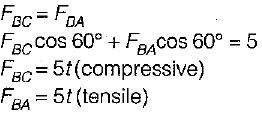QUESTION: 6

A simply supported truss shown in the given figure carries a load of 20 kN at F the forces in the members EFand BE are respectively: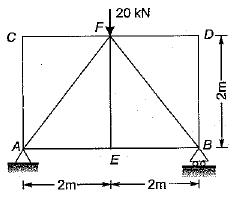Solution:

Force in EF is zero if joint equilibrium at E is considered force in SE will be tensile, and its value will be 10 kN by considering joint equilibrium at B.

QUESTION: 7

Axial force in the member BC of the truss shown in the given figure is (where α = 30°)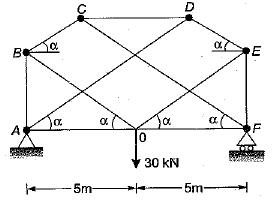Solution:

The given truss is symmetrical. So force in member BO and EO will be same in magnitude and nature both. Joint equilibrium at O.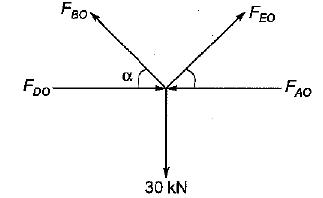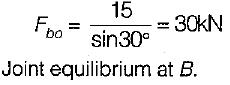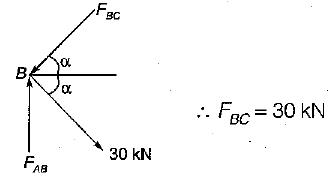QUESTION: 8

Axial forces in the members 1-2 and 1 - 5 of the truss shown in the given figure .are respectively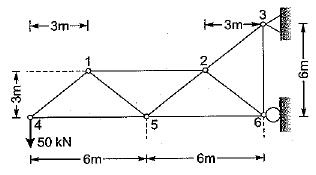Solution:

Cutting a section through 1-2; 2-5; and 5-6. Taking moment of left part about 5,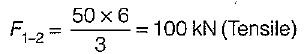Cut a section through 1-2, 1-5 and 4-5 and balance vertical force for left part only,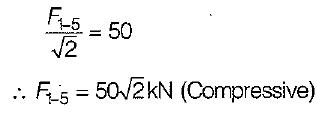QUESTION: 9

A pin-jointed tower truss is loaded as shown in the below figure. The force induced in the member DF is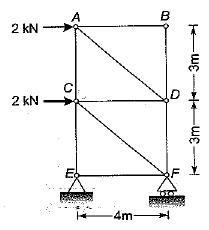Solution:

Cutting a section through AC, CD and DF and taking moment of upper portion about C.The forces in the members AC and CD meet at C so they do not produce any moment. The force in member DF,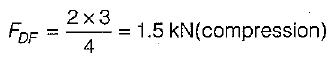QUESTION: 10

For the truss shown in the figure, which one of the following members has zero force induced in it?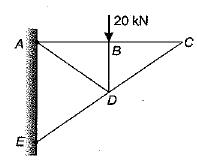Solution: